### Arizona Desert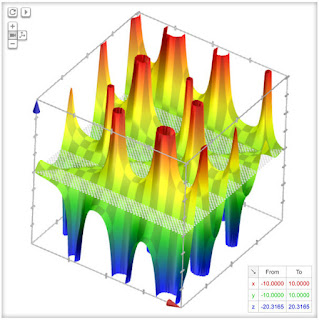z = (x-y)/(sin(x)+1/cos(y))

-

Reminds me of Sedona, and all the westerns filmed there.

### Bamboo ForestDensity plot: z = sin(2x)-cos(x/2)+tan(x)

-

It took a long time to find just the right zoom on this one.

Notice the extreme scale. In the end, I went north of 10^120 before being satisfied that I'd found the best possible zoom.

Apparently, working with numbers 100 times larger than a trillion-trillion-google was a bit too much for the software. I broke Grapher in the process, and will have to reinstall it.

Worth it!

### Bed o' Nailsz = sin(4y)+cos(4x)

-

Looks like nails. Actually, this is the simple egg carton plot graphed at a different scale.

### Blue Signal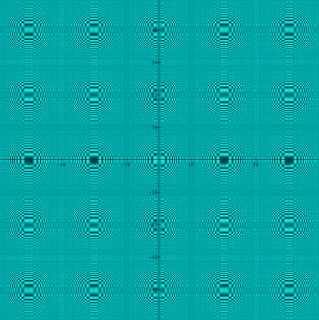Density plot: z = sin(x)^2*cos(y)^2

-

Simple expressions with interesting graphs are the best.

"Things should be as simple as possible, but no simpler."

### Bubbling Mesa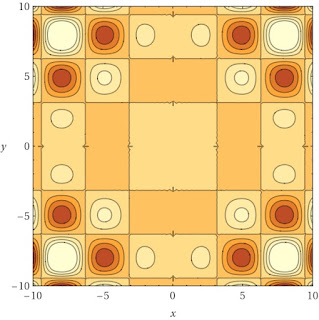Graph and contour plot: z = xysin(x)sin(y)

-

Dynamic, dream-like. Contour plot reveals multiple symmetries.

### Carpetrtan(sin(x)+cos(y))^2 < xlog(y)

-

Reminds me of a rug design, somehow.

### Celery Rowz = sin(x)cos(y)/(x-y)

-

Mathematical veggies.

### Cells Under MagnificationDensity plot: z = sin(ncos(x+y)/tan(xy))

-

Note the intricate complexity of material within cell walls (like real cells).

### Convergencey = kx/3
k = {1..18}

-

Dirt-simple linear equations. Interesting effect.

[Idea borrowed from another math artist: https://samjshah.com/2013/01/08/families-of-curves/]

### Corrugated V-Roof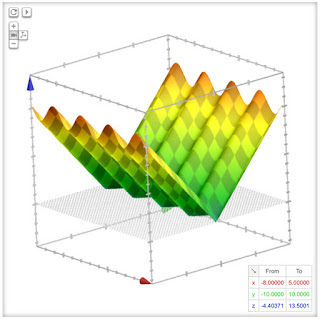z = abs(sin(2x)-y)

-

Like the inexpensive, corrugated metal roofs covering many Balinese homes, including that of my in-laws.

### Diffraction 1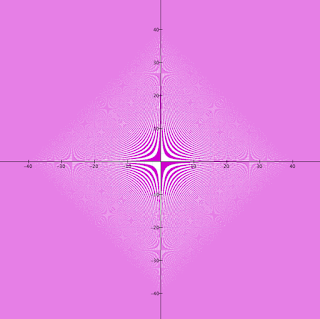cot(sin(xy)) < tan(cos(xy))

-

Interesting diffraction effects caused by the limited resolution of the graphing tool.

### Diffraction 3Density plot: z = 0.5sin(xyarctan((x/y)tan(x/y)))

-

A complex expression. Zooming out creates a series of interesting diffraction patterns.

### Dimpled Vasez^2 = x^6-x^2+y^6+y^2

-

I was utterly surprised by this plot. Reminds me of ceramic art.

### Double-Backr = t^2-t
𝜃 = tsin(t)
t = {0..40}

-

Intriguing variation on the spiral theme.

### Dripping Painttan(.5ycos(x)) ≤ -cos(sin(y-2x))

-

I owned and operated a house painting business as a young man. Before sprayers, it was all rollers and brushes. Dried drips were a scourge.

Shaded areas of the plot above were too complex for Desmos to graph. Makes a nice effect.

### Egg Cartonz = sin(x/2)+cos(y/2)

-

Who doesn't like eggs?

### Egg Carton ContoursContour plot: z = tan(xy)sin(y)cos(x)

-

4-up collage of egg carton contour plots.

### Electric Rain 1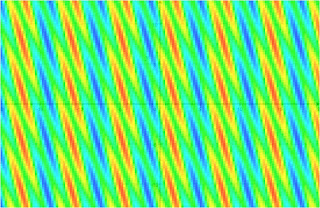Density plot: z = sin(x)+cos(3x+y)

-

One problem with Grapher is that it's a bit unstable. Changing window dimensions will force a recalculation, and you end can end up with a completely different picture, unable to return to the original.

Such was the case with this relation, but I ended up with a couple of nice plots, anyway.

### Electric Rain 2Density plot: z = sin(x)+cos(3x+y)

-

A different zoom.

### Eye of Redcos(ry^2+x^2) < 0

-

Had to use a close zoom, otherwise the shimmering effect was too annoying. As it is, it's just stimulating enough.

### Fender Butterflyr = sec(x)
y = tan(x)+cot(x)+k
k = {-5..5}

-

Redolent of butterflies and Fender guitars.

### Laser-Etched LPDensity plot: z = sin(x)^2-cos(y)^2

-

Reminds me of the laser-etched version of the True Colours LP released by New Zealander pop group Split Enz in 1980.

An awesome record, both musically and physically. When light shined on the rotating lp, the reflected laser-etched designs would dance around the room.

### Lawn Chairz = x^2-y^5+cos(arcsin(cos(y)+sin(x)))

-

Add a simple frame, and this would make a fine garden chair, with holes to enhance air circulation.

### Line Dancing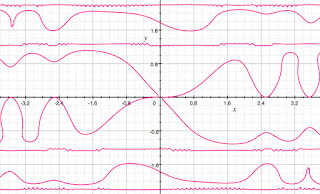cos(x^2)+sin(xy)+tan(y^2) = 1

-

Nice movement. Elusive symmetry.

### Line-UpDensity plot: z = -sin(ycos(x))

-

Also looks good in green.